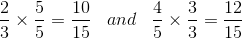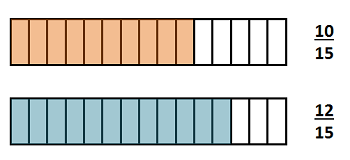# Comparing Fractions with Unlike Denominators

Instructor: Nola Bridgens

Nola has taught elementary school and tutored for four years. She has a bachelor's degree in Elementary Education, a master's degree in Marketing, and is a certified teacher.

How can we compare fractions that don't share the same denominator? By finding a common denominator for each fraction! In this lesson, you will learn how to find the least common denominator which will then allow you to compare fractions with unlike denominators.

## Unlike Denominators

Fractions consist of a numerator and a denominator. The numerator is the top number of a fraction that tells how many pieces of the whole we are talking about. The denominator is the bottom number of a fraction and it tells how many total pieces make up one whole. How do you compare fractions when they don't have the same denominator? You have to find common denominators.

## Common Denominators

A common denominator is a multiple that both denominators share. To compare fractions with unlike denominators, you need to find the least common denominator (LCD), or the smallest multiple the denominators share.

So, what fraction of your favorite candy bar would be more, 2/3 or 4/5? The two fractions have different denominators, so we need to find the LCD.

The first step is to list the multiples of each denominator:

Multiples of 3: 3, 6, 9, 12, 15, 18

Multiples of 5: 5, 10, 15, 20

The first multiple both 3 and 5 share is 15, so we need to make equivalent fractions with denominators of 15.

## Equivalent Fractions

Equivalent fractions are fractions that represent the same part of the whole. To find an equivalent fraction, you multiply the numerator and the denominator by the same number.

In our example, we need to make 2/3 and 4/5 both have a denominator of 15. We can multiply 2/3 by 5/5 and 4/5 by 3/3 to find equivalent fractions with denominators of 15.Now that both fractions have the same denominator, we can easily compare them.

## Comparing Fractions

When fractions have the same denominator, the larger fraction is the one with the larger numerator. If the numerators are the same, the fractions are equivalent fractions.

In our example, 12/15 is larger than 10/15, so 4/5 of the candy bar would be more. You would tell your mom that you would like 4/5 of your favorite candy bar, please.

The image shows what 10/15 and 12/15 look like.To unlock this lesson you must be a Study.com Member.

### Register to view this lesson

Are you a student or a teacher?

#### See for yourself why 30 million people use Study.com

##### Become a Study.com member and start learning now.
Back
What teachers are saying about Study.com

### Earning College Credit

Did you know… We have over 200 college courses that prepare you to earn credit by exam that is accepted by over 1,500 colleges and universities. You can test out of the first two years of college and save thousands off your degree. Anyone can earn credit-by-exam regardless of age or education level.